# RBSE Class 9 Science Chapter 3: Atomic Structure Important Textbook Questions and Solutions

RBSE Class 9 Science Chapter 3 important questions and solutions help students to overcome their fear of examination. These textbook solutions are solved by subject matter experts, focusing on detailed explanations of each question. All the questions are solved accurately with proper examples and diagrams. Students should take the help of these RBSE Class 9 solutions of Chapter 3 while preparing for their Class 9 exam.

Class 9 Science Chapter 3 explains about atoms, molecules, protons, atomic weight, istotypes, etc. The Chapter will teach students different formulas to calculate mass number, atomic weight of an element and many more concepts related to atomic structure. Students should be thorough with their chapter so that they can answer all the questions confidently. In this article, we have provided complete solutions for Chapter 3.

### RBSE Class 9 Science Chapter 3: Objective Textbook Questions and Solutions

Q1. The Plum Pudding Model of Atom was given by

(A) Neil Bohr

(B) Thomson

(C) Ernest Rutherford

(D) Goldstein

Q2. The discovery of neutron was

(A) CV Raman

(B) Rutherford

(C) JJ Thomsan

Q3. The size of an atom is

(A) 10-6cm

(B) 10-15cm

(C) 10-2cm

(D) 10-8cm

Q4. the number of neutrons in the Deutirium Isotope of hydrogen is /are

(A) One

(B) Two

(C) Three

(D) Not even one

Q5. What is the Atomic Number of Hydrogen?

(A) 1

(B) 2

(C) 3

(D) 4

Q6. Which scientists invented the Atomic Theory in 1808?

(A) JJ Thomson

(B) John Dalton

(C) Ernest Rutherford

(D) Neil Bohr

Q7. The accepted unit of atomic and molecular masses is

(A) Pound

(B) Gram

(C) Atomic Mass Unit

(D) Kilogram

Q8. What are the particles present in the nucleus?

(A) Neutron and Electron

(B) Only Neutron

(C) Proton and Electron

(D) Proton and Neutron

Q9. Ammonium Phosphate contains how many elements?

(A) 4

(B) 5

(C) 3

(D) 2

Q10. Who suggested the presence of the nucleus at the centre of the atom?

(A) Rutherford

(B) Goldstein

(C) Bohr

(D) JJ Thomson

Q11. What is the correct electronic configuration of sodium atom?

(A) 1,2,8

(B) 8,2,1

(C) 2,8,1

(D) 3,6

Q12. Who discovered neutrons?

(A) Rutherford

(B) Goldstein

(D) JJ Thomson

Q13. All the matter is composed of small particles called

(A) Atom

(B) Electron

(C) Neutron

(D) Molecule

Q14. The mass of proton is how much greater than that of the electron?

(A) 1886

(B) 1884

(C) 1837

(D) 1844

Q15. Mass number is represented by.

(A) A

(B) Z

(C) n

(D) M

### RBSE Class 9 Science Chapter 3: Very Short Answer Type Questions and Solutions

Q16. What are Isotopes?

Answer: Atoms of the same element whose atomic number is the same but mass number is different are known as isotopes. For example: the two isotopes of carbon are Carbon-12 and Carbon-14

Q17. What are Isobar Element?

Answer: Isobars are the atoms of different elements whose mass number is the same but atomic number differs. For example: The mass number of calcium and argon, both, is 40 but the atomic numbers are 18 and 20, respectively.

Q18. Write the names of the basic particles present in atoms.

Answer: The basic particles present in atoms are Electron, Proton and Neutron.

Q19. Define Atomic weight.

Answer: The atomic weight of an element is that number which indicates how heavy is the atom of the element, as compared to the hydrogen atom.

For example: Atomic weight of an element = Weight of one atom of the element/Weight of 1/12 part of the C-12 isotope.

Q20: What is Atomic Number?

Answer: The number of protons present in an atom is known as the atomic number. It is denoted by Z.

Q21. What is the charge on neutron?

Answer: Neutrons don’t carry any charge. It is a neutral particle.

Q22. Write the value of Avogadro number.

Q23. Write the name of the discoverer of proton.

Answer: The proton was discovered by Goldstein.

Answer: Metallic radius is half of the total distance between the nuclei of the two adjacent atoms in a metallic cluster. This is its atomic radius.

Answer: Covalent radius is half the distance of single covalent bond formed between similar atoms. For example half of the distance between the nuclei of two chlorine atoms is 99Aº which is considered to be its atomic radius (1Aº = 10–8 cm).

Q26. What is the formula to determine the maximum number of electrons in a shell?

Answer: The formula to determine the maximum number of electrons in a shell is 2n2.

Q27. Name three types of Hydrogen Isotopes?

Answer: The three isotopes of hydrogen are Protium, Deutirium and Tritium.

Q28. What is the NTP volume of 1 mole of a gas?

Answer: The NTP volume of 1 mole of a gas is 22.4 litres.

Q29. What are electrons?

Answer: The negatively charged particles in the atom are electrons.

Q30. Who discovered neutrons?

### RBSE Class 9 Science Chapter 3: Short Answer Type Questions and Solutions

Q31: What is immersion tube? Explain with the help of a diagram.

Answer: Immersion tube is a long glass tube having metal electrode on both ends. A vacuum pump is connected to it, with the help of which the pressure in the tube can be increased or decreased. With its help even vacuum can be created in the tube.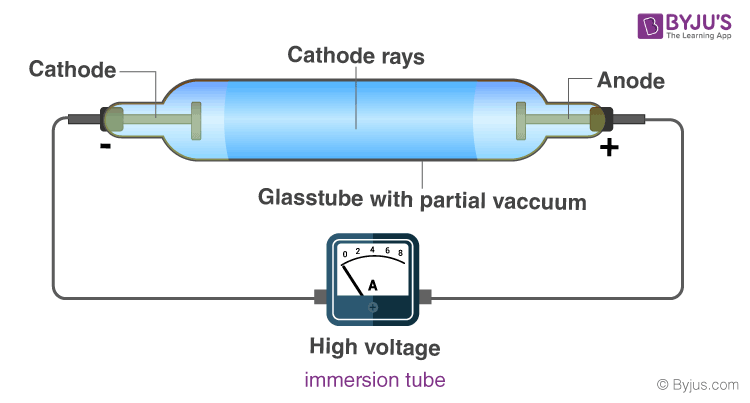Q32. Explain the atomic model of Thomson.

Answer: J.J.Thomson, in 1898, gave a visual picture of an atom. Having proved that electrons are fundamental negatively charged particles of an atom, he proposed the necessity of an equal number of positive charges, to make the atom neutral in nature. Combining the known facts he proposed an atom as a solid sphere of positive charge containing electrons on it. For example: water melon. The fleshy part of which is the positive region and the seeds present in it may be considered the electron. This model was named as the ‘ Plum Pudding Model ‘. Read more by clicking the link.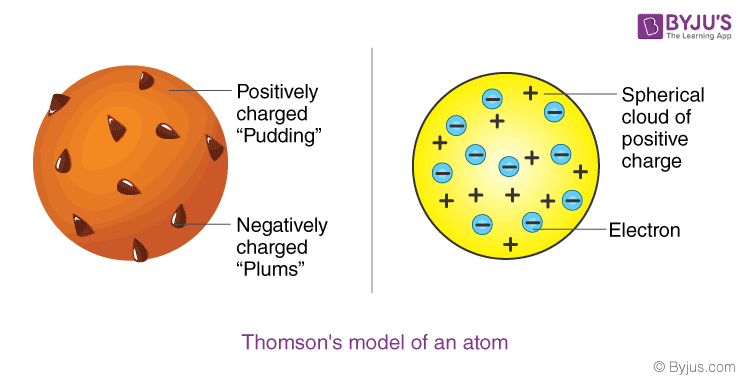Q33. What is mole concept? Explain.

Answer: According to the mole hypothesis “The mass of one mole of a substance is equal to its gram atomic weight or the gram molecular weight.”

According to this definition:

• The weight of 1 mole of the substance
• 18 gms water (H2O)
• 17 gms ammonia (NH3)
• 44 gms carbon-di-oxide (CO2)
• 12 gms carbon (C)
• 24 gms magnesium (Mg)

In one mole of every substance, the number of its particles (atoms, ions, molecules) is definite, which is known as the Avogadro number and its value is 6.022×10,23.

Q34. Write the main points of the Dalton’s Atomic Theory.

Answer: The main points of the Dalton’s Atomic Theory are;

• Atoms are tiny particles of which elements are made up of.
• All atoms present in a given element are identical.
• Atoms of different elements differ from one another.
• Compounds are formed by combining atoms of one element with atoms of other elements.
• In a given compound it always has the same relative numbers of types of atoms.
• Atoms can be divided into smaller particles, but it cannot be created, nor destroyed in the chemical process.
• A chemical reaction simply changes the way atoms are grouped together.

Q35. Write the characteristic properties of Cathode Rays.

Answer: The characteristics properties of Cathode Rays are:

• They move in a straight line.
• They produce fluorescence.
• They are affected by electric and magnetic fields.
• When they pass through a gas, they ionise it.
• They are made up of negatively charged particles.
• The e/m (charge/mass) i.e. ratio of charge to mass of these particles is uniform.
• Primarily they are the matter waves.

Q36. Explain the Covalent Radius of atom with the help of an example.

Answer: Covalent radius is half the distance of single covalent bond formed between similar atoms. For example half of the distance between the nuclei of two chlorine atoms is 99Aº which is considered to be its atomic radius (1Aº = 10-8 cm).

### RBSE Class 9 Science Chapter 3: Essay Type Questions and Solutions

Q37. Elucidate the atomic model based on Rutherford’s observations of Gold foil particle scattering experiments.

Answer: Ernest Rutherford bombarded a thin screen, i.e. foil of gold encoated with zinc sulphide, with alpha particles. The following observations were made by this experiment:

• Most of the particles went out straight, without scattering.
• Some particles scattered at an angle of 90º and some at 120º angle.
• One out of 20,000 particles, however scattered at an angle of 180º i.e. returned on the same path after colliding with the foil.

Rutherford proposed the nuclear atomic model. The main points of this model are :

• The entire positive charge and mass of an atom is concentrated in a small part, the nucleus, at its center. The radius of the nucleus is 10–15 meter.
• Major part of the atom is void, in which the negatively charged electrons revolve at high speed on circular path, around the nucleus. These circular paths are known as orbit or shell or orbital.
• The ‘electrostatic force of attraction’ between the positively charged nucleus and the negatively charged electrons is balanced by the centrifugal force of the electrons revolving at high speed.
• The atom is electrically neutral because the total negative charge on electrons is equal to the total positive charge of the nucleus.

Q38. Write the main concept of the Neil Bohr atomic model and on its basis draw the atomic structure of sodium and potassium element.

Answer: Bohr’s model consists of a small nucleus (positively charged) surrounded by negative electrons moving around the nucleus in orbits. Bohr found that an electron located away from the nucleus has more energy, and electrons close to the nucleus have less energy.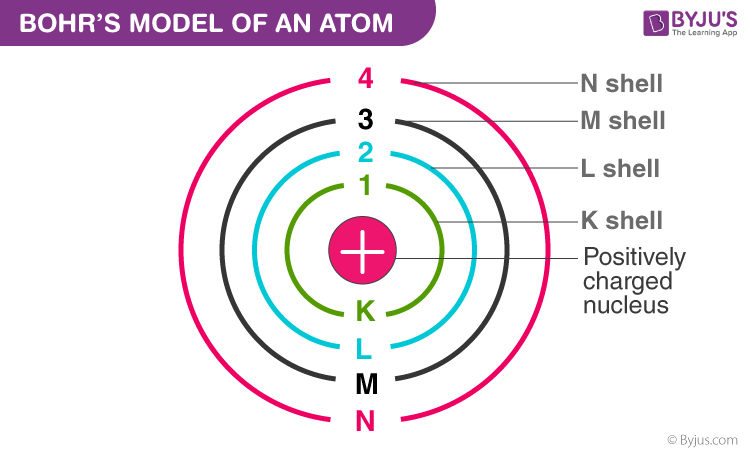Postulates of Bohr’s Model of an Atom

• In an atom, electrons (negatively charged) revolve around the positively charged nucleus in a definite circular path called orbits or shells.
• Each orbit or shell has a fixed energy and these circular orbits are known as orbital shells.
• The energy levels are represented by an integer (n = 1, 2, 3…) known as the quantum number. This range of quantum number starts from nucleus side with n = 1 having the lowest energy level. The orbits n = 1, 2, 3, 4… are assigned as K, L, M, N…. shells and when an electron attains the lowest energy level it is said to be in the ground state.
• The electrons in an atom move from a lower energy level to a higher energy level by gaining the required energy and an electron moves from a higher energy level to lower energy level by losing energy.

Sodium Atomic Structure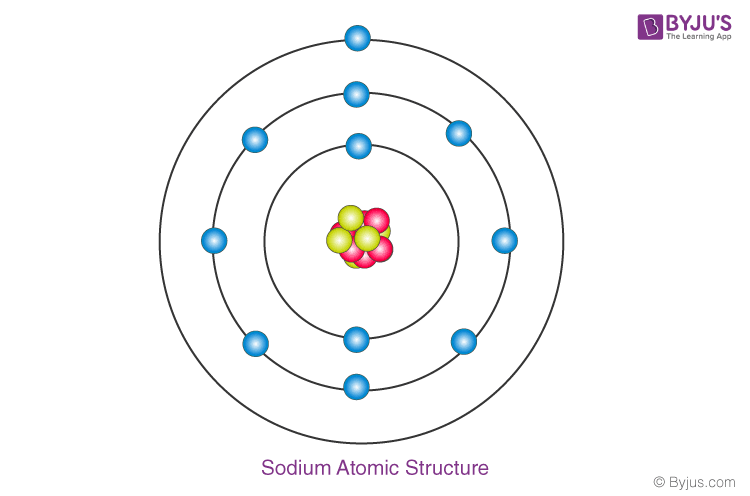Potassium Atomic Structure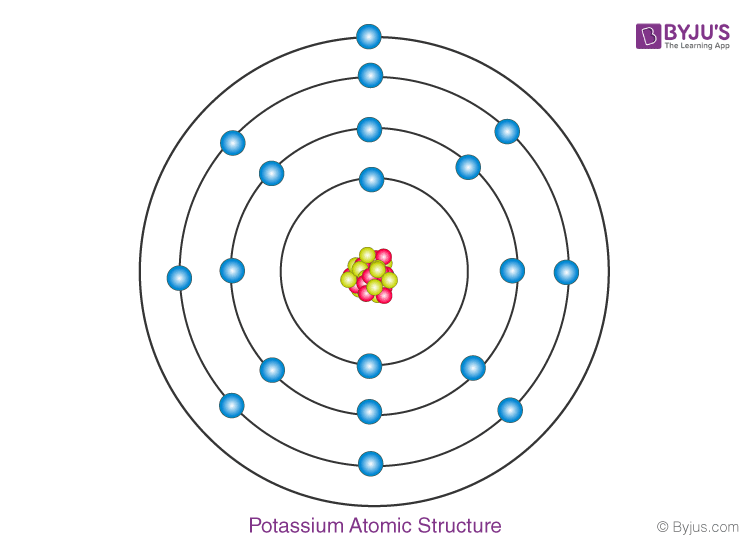Q39. What are positive rays? How can they be obtained? Write their properties.

Answer: In 1886, E. Goldstein used perforated cathode in the immersion tube and observed new type of rays at low pressure and high voltage, which were termed as positive rays. They are also known as the anode rays.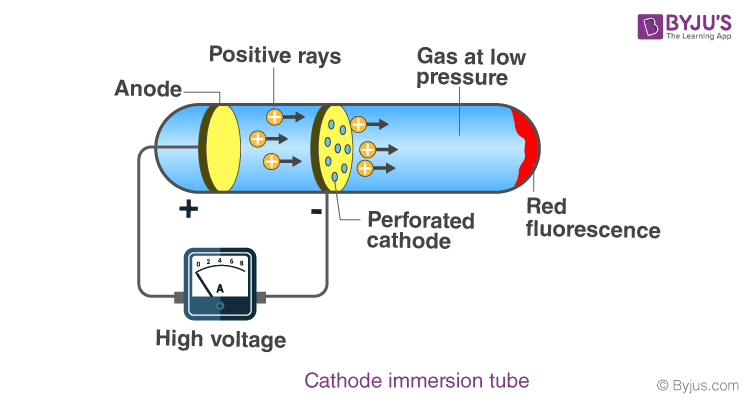Properties of the Positive rays :

• Positive rays move in a straight line.
• Their charge to mass ratio (charge/mass i.e. e/m) depends on the nature of gas present in the tube.
• They are affected by the electric and magnetic fields.
• They are made up of positively charged particles.
• They also are matter rays.

### RBSE Class 9 Science Chapter 3: Numerical Problems and Solutions

Q40. The number of neutrons in an isotope of an element is 9 and mass number is 17. Name the element and state its atomic number.

Answer: Mass number(A) can be calculated by using the formula: Z + n, where Z = Atomic number and n = Number of neutrons. So,

M = Z + n

17 = Z + 9

Z = 17 – 9

Z = 8

The Atomic number is 8 and the name of the element is oxygen.

Q41. Write the weight of 22.4 litre nitrogen at NTP in terms of gram.

Answers: The NTP volume of 1 mole of a gas is 22.4 litres. Nitrogen has a molecular weight of 28 grams.

Therefore, 22.4 litres of Nitrogen at NTP has weight = 28 grams.

Q42. How many atoms of carbon are present in 1.5 moles of carbon?

Answer: One mole of carbon (C) = 6.022 × 10,23

1.5 mole of carbon

= 6.022 × 10,23 × 1.5

= 9.033 × 10,23 atoms of carbon

Therefore, 9.033 × 10,23 atoms of carbon are present in 1.5 moles of carbon

Q43. What is the number of total atoms present in 9 grams of water.

Answer: We already know that 1 mole of any particular compound contains 6.022 × 1023

In the given question,

Mass of water = 9 gm

Mol. weight of water = 18

Hence, 9 gms would be 0.5 mole

1 mole contains 6.022 x 1023 atoms

So it follows (6.022 x 1023)/2 atoms are in 9 g of water.

Therefore, there are 9.033×1023 atoms